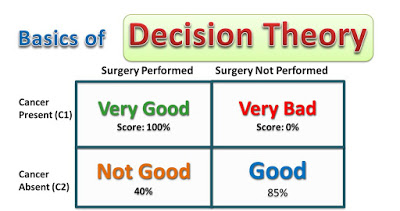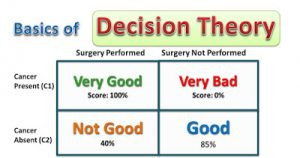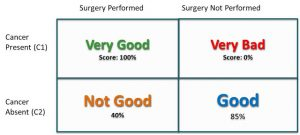October 28, 2021# Basics of Decision Theory – How Medical Diagnosis Apps Work

Watch the video here.

In this lesson we would examine the following topics

### 1. What is Decision Theory?

This is mathematical theory in the field of Machine Learning that allows us to make optimal decisions in situations involving uncertainty.### 2. Application of Decistion Theory in Cancer Diagnosis

Let’s illustrate decision theory using a medical situation where a physician needs to decide if a patient have cancer or not.

Now the physician request for an X-Ray of the patient so he can examine the film. He would pay attention to the intensity of the pixels in the image. which we would represent a x (input).

He have to determine an output t which would either be 1 (presence of cancer) or 0 (absence of cancer).

From his decision, he would take one of two actions, either perform a surgery, or not to perform a surgery. This is illustrated in the figure below:Let’s examine the scenarios

Scenario 1: There is presence of cancer and the physician decides to perform a surgery. That is 100% because its the best decision to take.

Scenario 2: There is presence of cancer but the physician decides not to perform a surgery. That is a score of 0 as it is the worst case scenario and of course the consequences would be very serious.

Scenario 3: Cancer is absent but the physician decides to perform a surgery anyway. This is a low score but does not result in any serious consequence

Scenario 4: Cancer is absent and the physician decides not to perform a surgery. This is a good decision as well.

### 3. The Goal of Decision Theory

So from the figure  we can see that the goal of the physician would be to get the highest score possible which is 100% and that is the objective of Decision Theory, to make the most optima decision.

### 4. Formal Definition of Decision Theory

Let’s not look at a formal definition of Decision Theory and we would pay attention to a few mathematical model, but I would try as much as I can to keep it simple.

Consider that we have an input vector x

A corresponding vector t of the target variables ( which could be 1 or 0)

And the two classes C1 and C2 (C1 = presence of cancer, C2 = absence of cancer)

Let t = 1 correspond to class C1 and

t = 0 correspond to class C2

The general inference problem is to determine the joint distribution p(x, Ck). Here k = 1,2. This is the same as as p(x,t). Decision theory is concerned with how to make optimal decisions given the appropriate probabilities.

In the next article we would go into a more details analysis.

### 5. False Positives and False Negatives

We take example of the cancer diagnosis example. Let’s assume that after a test the physician decides that based the diagnosis, cancer is present (that is a positive result is obtained for cancer test). If actually cancer is not present, then this result is known a false positive.

If on the other hand the doctor finds out that there is no cancer(a negative result is obtained) and actually there is cancer, then this result is considered false negative.

One objective of decision theory is to minimize both the false positive rate and the true positive rate.

### 6. Minimizing Misclassification and Reducing Expected Loss

When there is misclassification, a loss is incurred. Take for example, a patient image is classified as having cancer when actually there is not cancer. In this case, the loss would include cost incurred to perform a surgery, and the discomfort the patient experiences.

In the second case, a patient that actually have cancer is classified as not having cancer. The loss that would in incurred would be much worse as it may cost the life of the patient.

The aim is first to minimize misclassification and second to ensure tha the second type of loss is reduced or eliminated

### 7. What is Reciever Operating Characteristic(ROC) Curve

Receiver operating characteristic curve is a plot showing the diagnostic ability of a binary classifier system as its discrimination threshold is varied.

The ROC curve is created by plotting the true positive rate(TPR) against the false positive rate (FPR) at various threshold settings

### 8. Area Under the Curve(AUC)

The area under the curve  is equal to the probability that a classifier will rank a randomly chosen positive instance higher than a randomly chosen negative one.#### kindsonthegenius

View all posts by kindsonthegenius →
0 0 vote
Article Rating
Subscribe
Notify of1 Comment
Inline Feedbacks